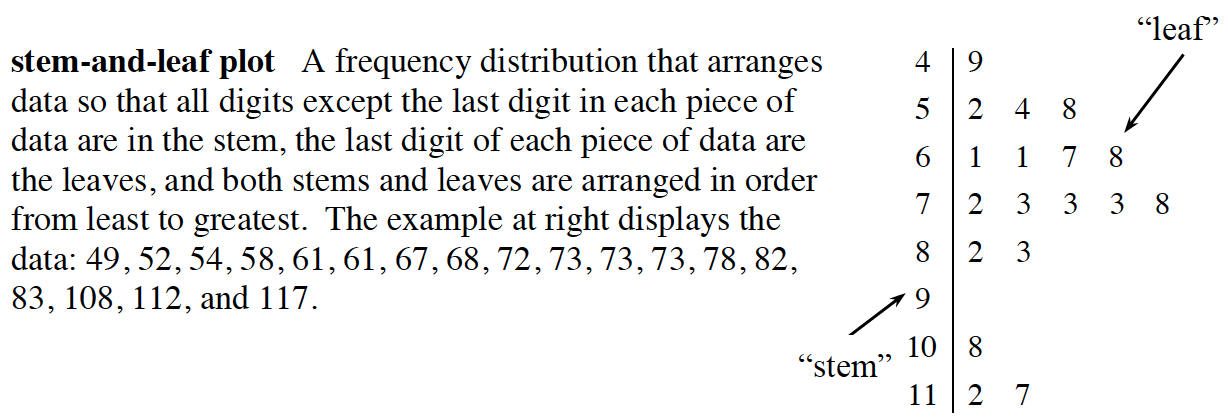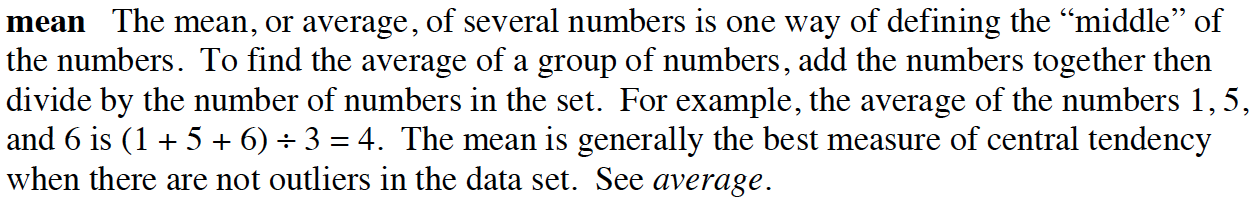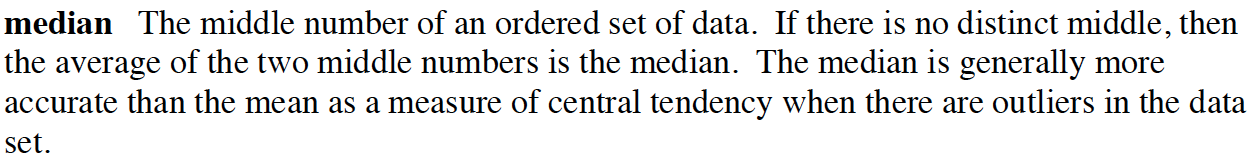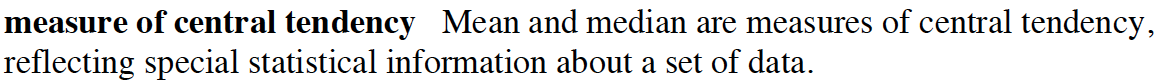### Home > CC3 > Chapter 1 > Lesson 1.1.4 > Problem1-38

1-38.

Consider this data: $22$, $15$, $30$, $51$, $27$, $33$, $19$. Homework Help ✎

1. Arrange the data into a stem-and-leaf plot. (Refer to the glossary if you need a reminder of what a stem-and-leaf plot is.)

2. Find the mean and median.

3. If the value $51$ was replaced with $33$, which measure(s) of central tendency would change and which would not? Explain.